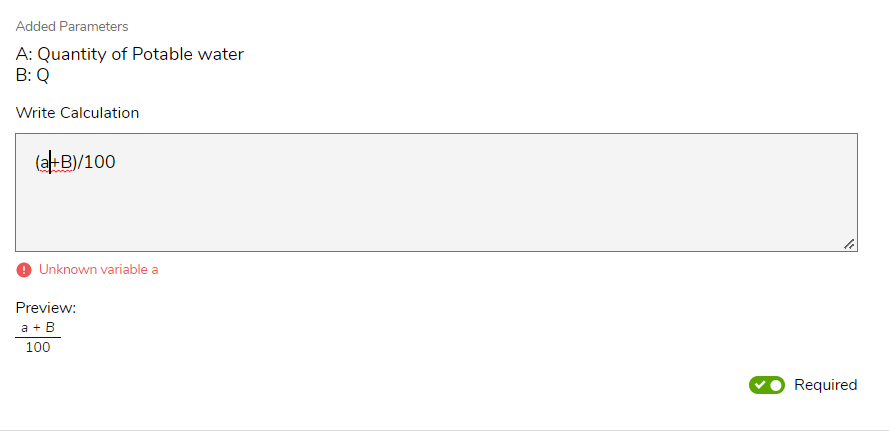## Users can add calculation activities that can be used to auto-calculate a quantity while executing a Job...

• Select Calculation activity from the list of 'Add Activity' dropdown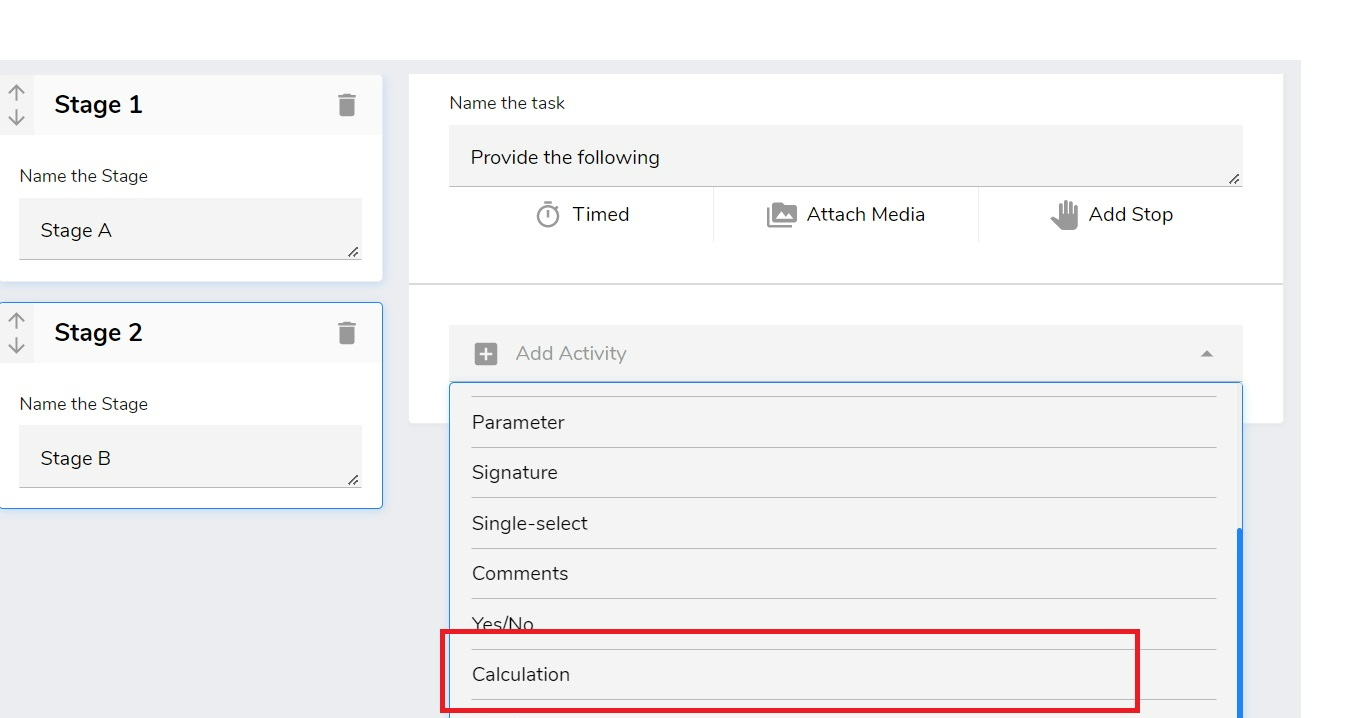• Enter the Label for the activity, description, and the Unit of measurement for the added calculation activity.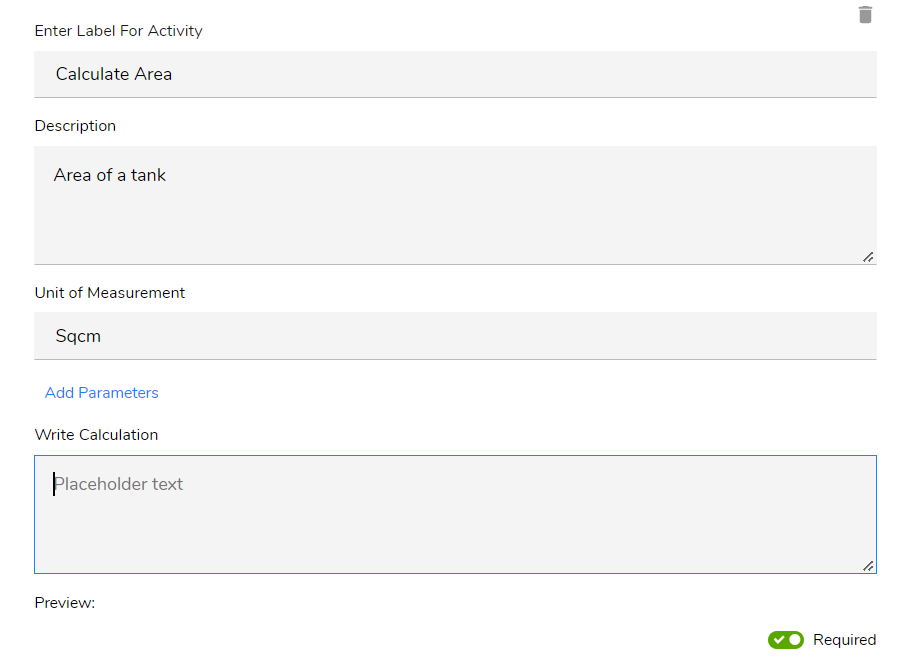• Users can add parameters that will be used as input data for the calculation2. Enter the name of the parameter and select a value for the parameter from the listed dropdown.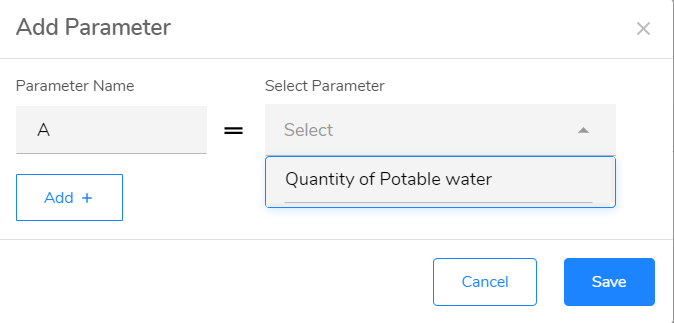3. To add multiple parameters Click on the Add+ button and you can configure multiple               parameters.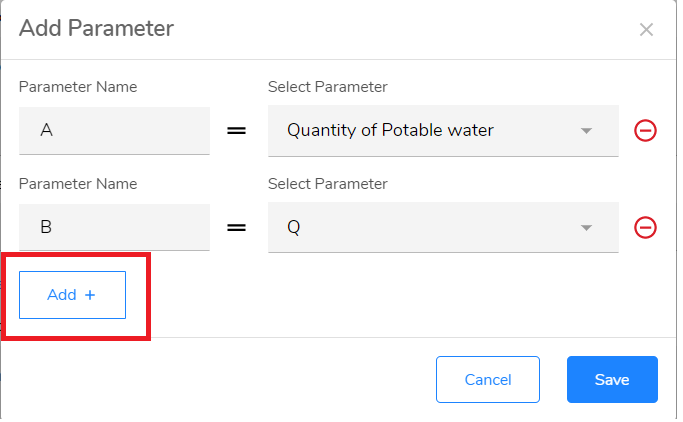4. Click on Save and the added parameters will be listed down and can now to used to                 configure a Formula.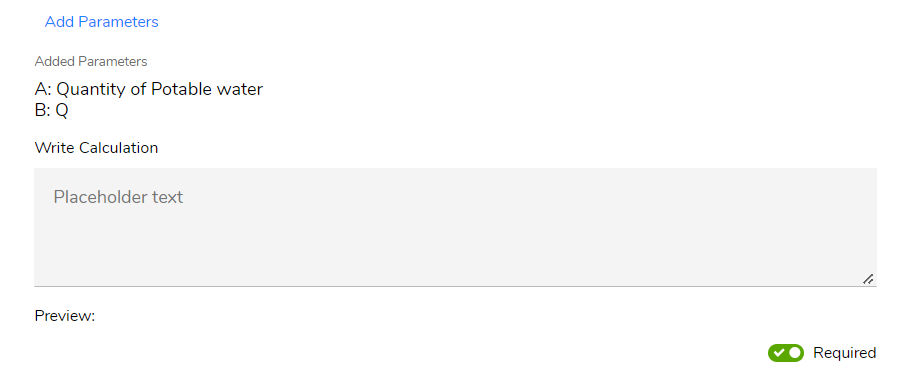• Write the required formula by using various mathematical operators in the Write Calculation box.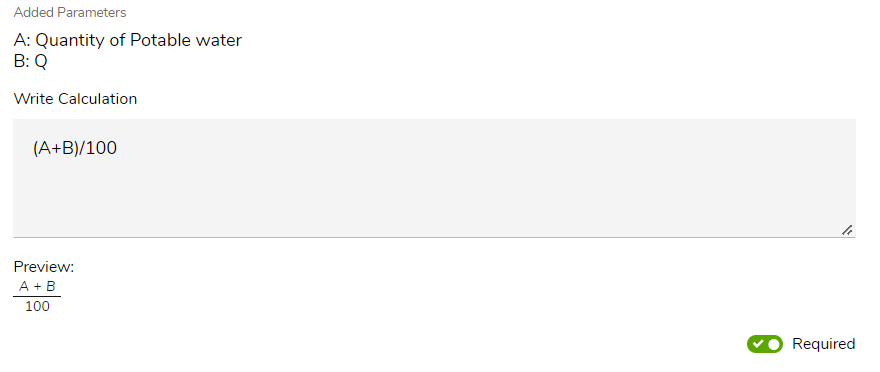• A preview of added formula will be displayed in the Preview section that will help you enter the correct formula and minimize the errors.

Note:
1. While writing a formula you can input only those variables which are available as the first column in the Added Parameters list. If you try to add a formula with a parameter other than in the available Added Parameters list, the system will throw an error as shown below: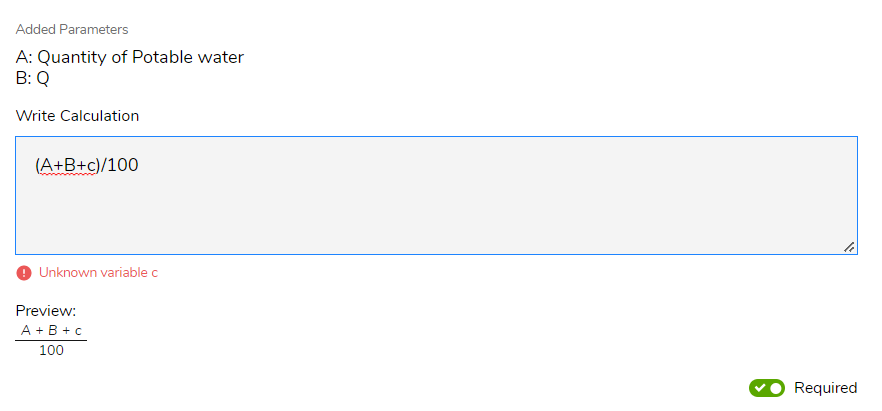2. Also the calculation box is case sensitive
for example, if you have added a parameter as A and your formula has an input of a, the system will show an error message as below: